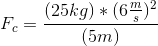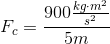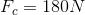# AP Physics B : Understanding Circular Motion

## Example Questions

### Example Question #1 : Understanding Circular Motion

A ball of mass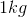on a string is rotating about the center axis with a velocity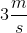. If the tension force in the string is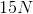, what is the radius of the circular path of the ball?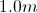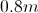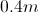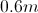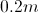Explanation:

We use the equation for centripetal force to find the radius: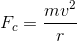Since the string ties the ball to the axis, the force of tension will be equal to the centripetal force.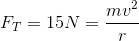Use the given mass and velocity to solve for the radius.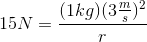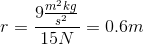### Example Question #2 : Understanding Circular Motion

A yo-yo trick called Around the World involves swinging the yo-yo around in a vertical circle at the end of its string. A man performs this trick with a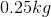yo-yo traveling at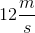. If the length of the full string is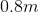, what is the centripetal force on the yo-yo?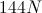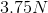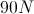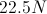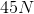Explanation:

Centripetal force can be found using the equation:In this situation, the radius is the length of the string. We are given the linear velocity and mass, as well as the radius, allowing us to solve for the centripetal force.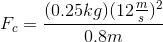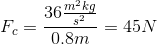### Example Question #4 : Centripetal Force And Acceleration

A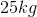boy is riding a merry-go-round with a radius of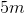. What is the centripetal force on the boy if his velocity is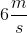?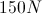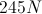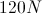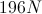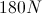Explanation:

For this problem, we use the centripetal force equation:We are given the mass, radius or rotation, and the linear velocity. Using these values, we can find the centripetal force.